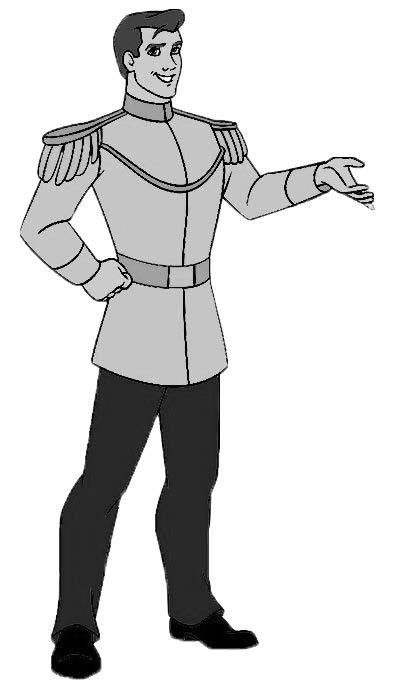# A Puzzle by Midnight MathImagine a game that involves betting on the color of a single card in a standard 52-card deck. Each card is turned over one by one, and before each card is flipped, you may do one of two things:

1) Bet: If the next card is red, you earn \$1. If the next card is black, you lose \$1.

2) Pass: The next card is turned over and shown to you, and play continues.

Once you bet on a card, the deck is reshuffled and may play again. If you reach the end of the deck, you are forced to bet on the last card.

In a naive strategy, you could bet on the first card of each deck, winning 50% of the time and earning, on average, \$0. Can you produce a better strategy, or a proof that one does not exist?

Send in your solutions (with proofs) to midnight.math@gmail.com or talk to Kevin O’Toole or Ian Hoover. If you are correct, you will be given the highest of accolades: your name mentioned here, next issue.

# A Puzzle by Midnight Math

New Puzzle
Try to imagine an irrational number between 0 and 1, represented in decimal, such that nowhere in the infinite sequence of digits does the same digit repeat twice in a row. Does such a number exist? If so, show why. If not, show why not.

# A Puzzle by Midnight MathNew Puzzle

A prince is surveying his kingdom. No matter where he stands, if he walks 100 paces forward in a straight line, turns to the left 90 degrees and walks 100 paces forward again in a straight line, again turns 90 degrees to the left and walks 100 more paces in a straight line, he arrives where he began walking. Where is he? (Bonus points, who is he?)

# A Puzzle by Midnight Math: May

The passel of math students from the first ever Midnight Math puzzle again find themselves held captive by that rascally hyper-intelligent, pan-dimensional being.

This time they are each held in separate cells and each day, one is chosen at random to come to a special room to work on a generalized integral transform problem (each selection is completely independent of any of the previous choices).

# A Puzzle by Midnight Math: April

Imagine you have n points evenly spaced around a circle. Choose one of these as the starting point, p0. Take a pen and draw a line skipping m – 1 points so that you connect p0 to pm.

Continue doing this without lifting the pen (so next you connect pm to the point m points later, and so on). Eventually you get back where you started.

Perhaps you have drawn an n-pointed star (the classic way of drawing a 5 pointed start is with n = 5 and m = 2).

Why can you never get a six pointed start this way? What other values of n will never result in a star?

# A Puzzle by Midnight Math: March

Let’s examine the following procedure: Start with a finite string of digits and replace each substring consisting of a repeated single digit with the number of digits in that substring followed by the digit of that that is being repeated. Example: 333 would become 33, and 2 would become 12 and 222233333 would become 4253. Starting with 1, and recursively applying this procedure generates the sequence: 1, 11, 21, 1211,… and so on. What is the largest digit that will ever appear in this sequence?

# A Puzzle by Midnight Math: FebruaryOn a circle are placed an equal number of “x”s and “o”s.

Starting with a value of zero, you chose a starting place on the circle and begin moving clockwise around the circle.

Every time you pass an “x”, you add 1 to your value, and every time you pass an “o” you subtract 1. Once you have returned to your starting location you stop.

Show that no matter how the “x”s and “o”s are placed (as long as there are an equal total number of each) there will always be a starting location such that your value is never less than zero.

# A Puzzle by Midnight Math: DecemberYou have a wire (of unit length) with a finite number of ants arbitrarily placed on it, each facing an arbitrary direction to start.

Each ant has negligible size and travels at the same, constant speed along the length of the wire.

When an ant reaches the end of the wire, it turns around instantaneously and continues moving.

# A Puzzle by Midnight Math: November

A puzzle, courtesy of the Midnight Mathematicians: “A passel of math students are kidnapped and held captive in some unknown, and probably unfriendly, vector space. On the forehead of each student, without their knowledge, is drawn either a 1 or a 0. The hyper-intelligent, pan-dimensional captor will only give the information necessary to leave said vector space to students who correctly identify the mark on their forehead. At the end of each day, each student has the opportunity to guess, but an incorrect guess means they will be trapped forever. The students are not allowed to communicate in any way, but every day they see each other for their one meal together (also, there are no surfaces which produce clean reflections in this space). The only information they are given is that there is at least one student with a 1 and at least one student with a 0. What is the largest number of days it could need to take for n students (n>=2) to all set themselves free?”